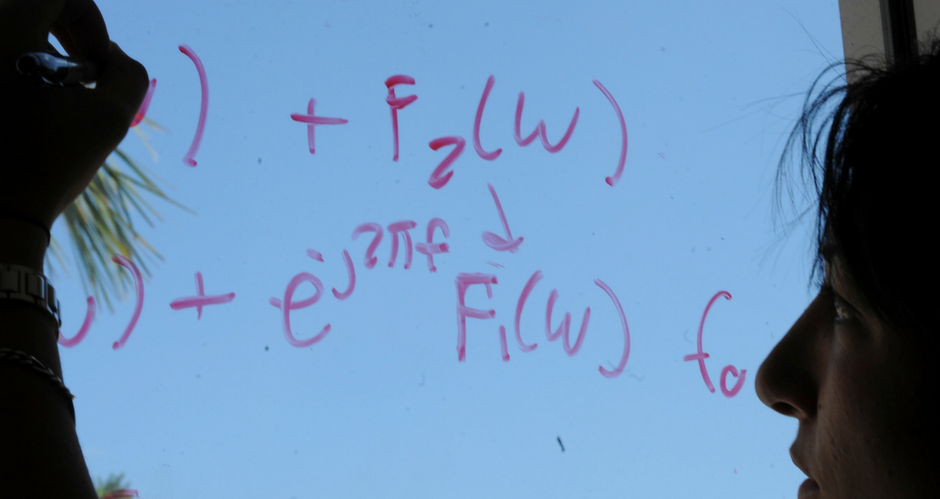## Mathematics and System Engineering Faculty Publications

### Philos-type oscillation criteria for second order half-linear dynamic equations on time scales

Article

#### Publication Title

Rocky Mountain Journal of Mathematics

#### Abstract

In this paper we establish some oscillation theorems for the second order half-linear dynamic equation (r(t)(x Δ(t) γ) Δ + p(t)x γ(t) = 0, ∈ [a,b], on time scales. Special cases of our results include some well-known oscillation results for second-order differential and half-linear differential equations. Our results are new for difference, generalized difference and q difference half-linear equations. Copyright © 2007 Rocky Mountain Mathematics Consortium.

1085

1104

#### DOI

10.1216/rmjm/1187453098

2007

COinS